Courses

Matrix-Match & Integer Answer Type Questions: Current Electricity | JEE Advanced Notes | Study Class 12 Physics 35 Years JEE Mains &Advance Past year Paper - JEE

JEE: Matrix-Match & Integer Answer Type Questions: Current Electricity | JEE Advanced Notes | Study Class 12 Physics 35 Years JEE Mains &Advance Past year Paper - JEE

The document Matrix-Match & Integer Answer Type Questions: Current Electricity | JEE Advanced Notes | Study Class 12 Physics 35 Years JEE Mains &Advance Past year Paper - JEE is a part of the JEE Course Class 12 Physics 35 Years JEE Mains &Advance Past year Paper.
All you need of JEE at this link: JEE

Each question contains statements given in two columns, which have to be matched. The statements in Column-I are labelled A, B, C and D, while the statements in Column-II are labelled p, q, r and s. Any given statement in Column-I can have correct matching with ONE OR MORE statement(s) in Column-II. The appropriate bubbles corresponding to the answers to these questions have to be darkened as illustrated in the following example :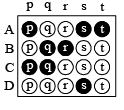If the correct matches are A-p, s and t; B-q and r; C-p and q; and D-s then the correct darkening of bubbles will look like the given.

Q.1. Column I gives some devices and Column II gives some processes on which the functioning of these devices depend. Match the devices in Column I with the processes in Column II and indicate your answer by darkening appropriate bubbles in the 4 × 4 matrix given  in the  ORS.

 Column I Column II (A) Bimetallic strip (p) Radiation from a hot body (B) Steam engine (q) Energy conversion (C) Incandescent lamp (r) Melting (D) Electric fuse (s) Thermal expansion of solids

Ans. A → s; B → q; C → p, q; D →q, r

Solution.

A → s

Reason : Bimetallic strip is based on thermal expansion of solids.

B → q

Steam engine is based on energy conversion.

C → p, q

Incandescent lamp is based on energy conversion and radiation from a hot body.

D → q, r

Electric fuse is based on melting point of the fuse material which is turn depends on the heating effect of current.

Q.2. When two identical batteries of internal resistance 1Ω each are connected in series across a resistor R, the rate of heat produced in R is J1. When the same batteries are connected in parallel across R, the rate is J2. If J1 = 2.25 J2 then the value of R in Ω is

Ans.  4

Solution. Cells connected in series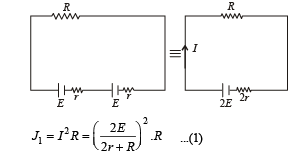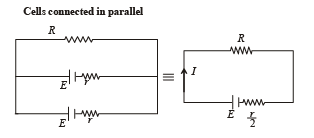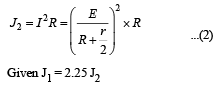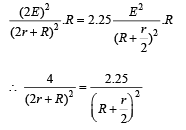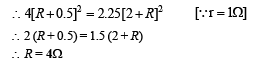Q.3. At time t = 0, a battery of 10 V is connected across points A and B in the given circuit. If the capacitors have no charge initially, at what time (in sceonds) does the voltage across them become 4 V? [Take : ln5 =1.6, ln3 = 1.1]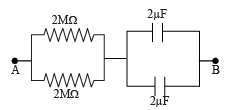Ans. 2

Solution. The equivalent circuit is shown in the figure.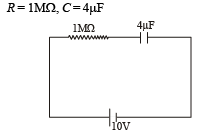∴The time constant τ = RC = 4 sec The potential across 4μF capacitor at any time ‘t’ is given as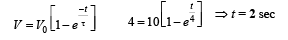Q.4. Two batteries of different emfs and different internal resistances are connected as shown. The voltage across AB in volts is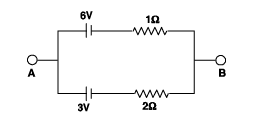Ans. 5

Solution. Let i be the current flowing in the circuit. Apply Kirchhoff’s law in the loop we get

–3 – 2i – i + 6 = 0

∴ 3i = 3

∴ i = 1 Amp

Now let us travel in the circuit from A to B through battery of 6V, we get

VA – 6 + 1 × 1 = VB

∴ V– VB = 5 volt.

Q.5.A galvanometer gives full scale deflection with 0.006 A current. By connecting it to a 4990 W resistance, it can be converted into a voltmeter of range 0 – 30 V. If connected to 2n/249Ω resistance, it becomes an ammeter of range 0 – 1.5A.The value of n is

Ans. 5

Solution.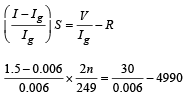∴n ≈ 5

Q.6. In the following circuit, the current through the resistor R (= 2 Ω) is I amperes. The value of I is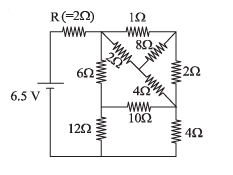Ans. 1

Solution. The equivalent resistance of balanced wheatstone bridge is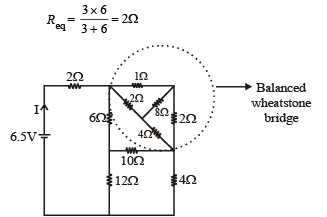The equivalent resistance of balanced wheat stone bridge is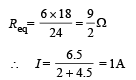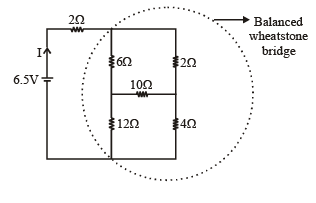The document Matrix-Match & Integer Answer Type Questions: Current Electricity | JEE Advanced Notes | Study Class 12 Physics 35 Years JEE Mains &Advance Past year Paper - JEE is a part of the JEE Course Class 12 Physics 35 Years JEE Mains &Advance Past year Paper.
All you need of JEE at this link: JEEUse Code STAYHOME200 and get INR 200 additional OFF Use Coupon Code

Top Courses for JEE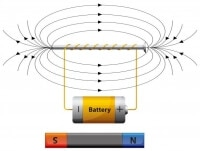Class 12 Physics 35 Years JEE Mains &Advance Past year Paper

42 docs|19 tests

Top Courses for JEETrack your progress, build streaks, highlight & save important lessons and more!

,

,

,

,

,

,

,

,

,

,

,

,

,

,

,

,

,

,

,

,

,

;